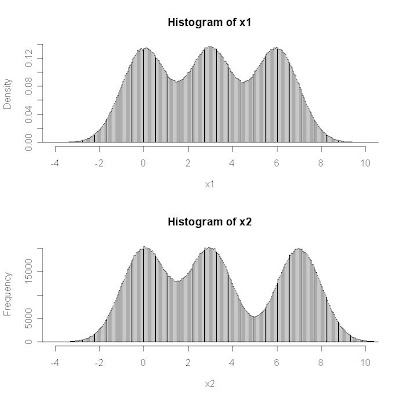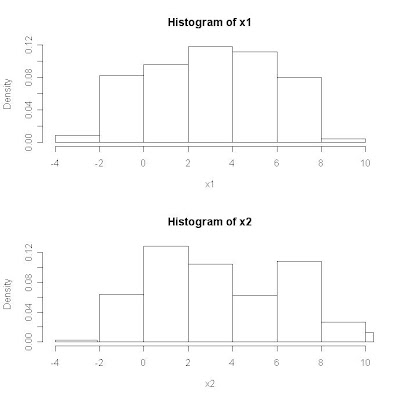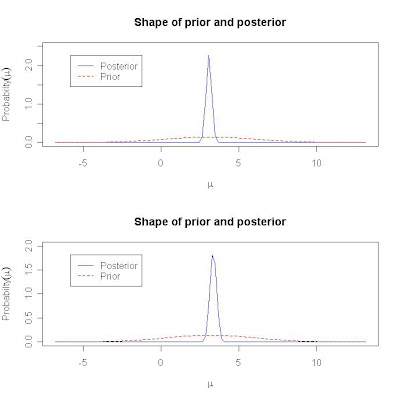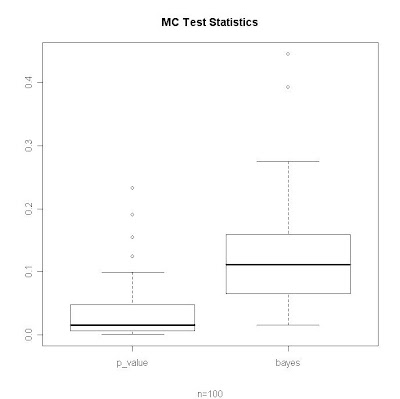### Being a Bayesian can be Difficult

Is sample 1 smaller than sample 2?

#### Population Distribution#### Sample n = 225#### Prior and Posterior for mu

I assume data is generated from a normal distribution and additionally assume a normal prior for mu. Set mu_prior = 3.166 and sd_prior = 2.85, the approximate true mean and standard deviation for the population.

Back out the Bayesian test probability as 1 - pr(mu_1 < mu_2) from numerical integration.#### MC Study: KS-test p-value vs. Bayesian Probability

Repeat the experiment n=100 times.